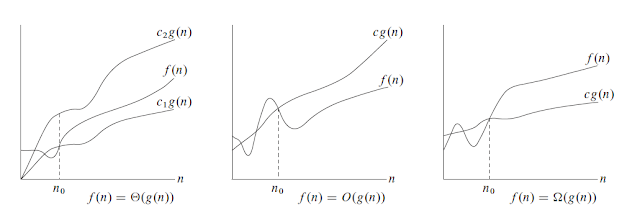# Asymptotic analysis of algorithms

`╔═════════════════╦═════════════╗║     Function    ║ Explanation ║╠═════════════════╬═════════════╣║ f(n) = c        ║ Constant    ║║ f(n) = log(n)   ║ Logarithmic ║║ f(n) = n        ║ Linear      ║║ f(n) = n.log(n) ║ Superlinear ║║ f(n) = n^2      ║ Quadratic   ║║ f(n) = n^3      ║ Cubic       ║║ f(n) = c^n      ║ Exponential ║║ f(n) = n!       ║ Factorial   ║╚═════════════════╩═════════════╝`Big theta vs Big oh vs Big omega

--

--